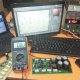Breaking News
Home / stk amplifier circuit diagram / STK430-443 circuit diagram

# STK430-443 circuit diagram

## STK430-443 circuit diagram:

This is the STK430-443 circuit diagram. this circuit is the stereo amplifier circuit diagram. It starting from 6+6 Watts to 28+28 Watts.

1. STK433 is 5watts maximum voltage is  DC 20.
2. STK435 is 7watts maximum voltage is DC 24.
3. STK436 is 10watts maximum voltage is DC 27.
4. STK437 is 12watts maximum voltage is DC 30.
5. STK439 is 16watts maximum voltage is DC 34.
6. STK441 is 24watts maximum voltage is DC 39.
7. STK443 is 28watts maximum voltage is DC 44.